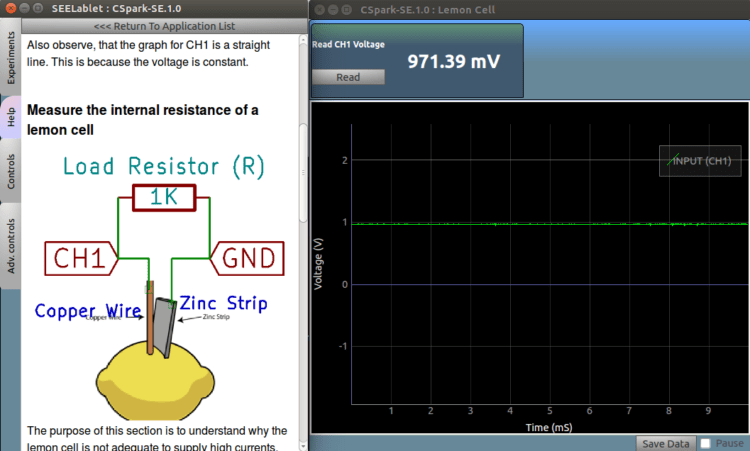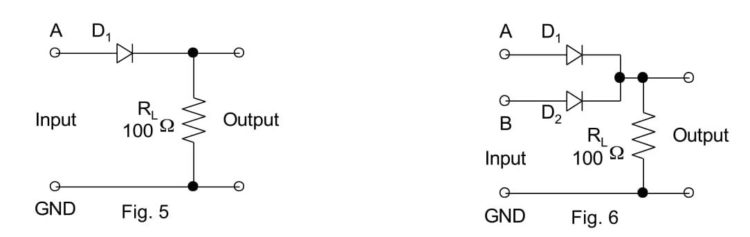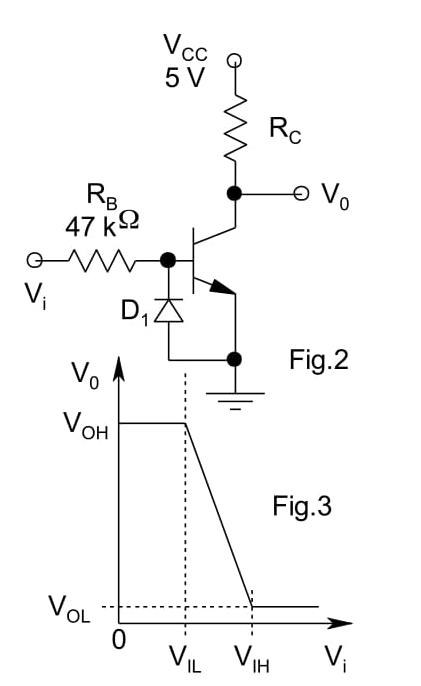## Fascinating Experiments with PSLab

PSLab can be extensively used in a variety of experiments ranging from the traditional electrical and electronics experiments to a number of innovative experiments. The PSLab desktop app and the Android app have all the essential features that are needed to perform the experiments. In addition to that there is a large collection of built-in experiments in both these experiments.

This blog is an extension to the blog post mentioned here. This blog lists some of the basic electrical and electronics experiments which are based on the same principles which are mentioned in the previous blog. In addition to that, some interesting and innovative experiments where PSLab can be used are also listed here. The experiments mentioned here require some prerequisite knowledge of electronic elements and basic circuit building. (The links mentioned at the end of the blog will be helpful in this case)

Op-Amp as an Inverting and a Non-Inverting AmplifierThere are two methods of doing this experiment. PSLab already has a built-in experiment dedicated to inverting and non-inverting amplification of op-amps. In the Android App, just navigate to Saved Experiments -> Electronics Experiments -> Op-Amp Circuits -> Inverting/ Non-Inverting. In case of the Desktop app, select Electronics Experiments from the main drop-down at the top of the window and select the Inverting/Non-inverting op-amp experiment.

This experiment can also performed using the basic features of PSLab. The only advantage of this methodology is that it allows much more tweaking of values to observe the Op-Amp behaviour in greater detail. However, the built-in experiment is good enough for most of the cases.

• Construct the above circuits on a breadboard.
• For the amplifier, connect the terminals of CH1 and GND of PSLab on the input side i.e. next to Vi and the terminals of CH2 and GND on the output side i.e next to Vo.
• Usually, an Op-Amp like LM741 have a set of pins, one dedicated for the inverting input and the other dedicated for the non-inverting input. It is recommended to consult the datasheet of the Op-Amp IC used in order to get the pin number with which the input has to be connected.
• The terminals of W1 and GND are also connected on the input side and they are used to generate a sine wave.
• The resistors displayed in the figure have the values R1 = 10k and R2 = 51k. Resistance values other than these can also be considered. The gain of the op-amp would depend on the ratio of R2/R1, so it is better to consider values of R2 which are significantly larger than R1 in order to see the gain properly.
• Use the PSLab Desktop App and open the Waveform Generator in Control. Set the wave type of W1 to Sine and set the frequency at 1 kHz and magnitude to 0.1 V. Then go ahead and open the Oscilloscope.
• CH1 would display the input waveform and CH2 will display the output waveform and the plots can be observed.
• If the input is connected to the inverting pin of the op-amp, the output obtained will be amplified and will have a phase difference of 90o with the input waveform whereas when the non-inverting pin is selected, the output is just amplified and no such phase difference is observed.
• Note: Take proper care while connecting the V+ and V- pins of the op-amp, else the op-amp will be damaged.

Diode as an Integrator and DifferentiatorAn integrator in measurement and control applications is an element whose output signal is the time integral of its input signal. It accumulates the input quantity over a defined time to produce a representative output.

Integration is an important part of many engineering and scientific applications. Mechanical integrators are the oldest application, and are still used in such as metering of water flow or electric power. Electronic analogue integrators are the basis of analog computers and charge amplifiers. Integration is also performed by digital computing algorithms.

In electronics, a differentiator is a circuit that is designed such that the output of the circuit is approximately directly proportional to the rate of change (the time derivative) of the input. An active differentiator includes some form of amplifier. A passive differentiator circuit is made of only resistors and capacitors.

• Construct the above circuits on a breadboard.
• For both the circuits, connect the terminals of CH1 and GND of PSLab on the input side i.e. next to input voltage source and the terminals of CH2 and GND on the output side i.e next to Vo.
• Ensure that the inverting and the non-inverting terminals of the op-amp are connected correctly. Check for the +/- signs in the diagram. ‘+’ corresponds to non-inverting and ‘-’ corresponds to inverting.
• The terminals of W1 and GND are also connected on the input side and they are used to generate a sine wave.
• The resistors displayed in the figure have the values R1 = 10k and R2 = 51k. Resistance values other than these can also be considered. The gain of the op-amp would depend on the ratio of R2/R1, so it is better to consider values of R2 which are significantly larger than R1 in order to see the gain properly.
• Use the PSLab Desktop App and open the Waveform Generator in Control. Set the wave type of W1 to Sine and set the frequency at 1 kHz and magnitude to 5V (10V peak to peak). Then go ahead and open the Oscilloscope.
• CH1 would display the input waveform and CH2 will display the output waveform and the plots can be observed.
• If all the connections are made properly and the values of the parameters are set properly, then the waveform obtained should be as shown below.

Performing experiments involving ICs (Digital circuits)

The experiments mentioned so far including the ones mentioned in the previous blog post involved analog circuits and so they required features like the arbitrary waveform generator. However, digital circuits work using discrete values only. PSLab has the features needed to perform digital experiments which mainly involve the use of a square wave generator with a variable duty cycle.

PSLab board has dedicated pins named SQR1, SQR2, SQR3 and SQR4. The options for configuring these pins is present under the Advanced Control section in the Desktop app and in the Android app Applications->Control->Advanced. The options include selecting the pins which we want to use for digital outputs and then configuring the frequency and duty cycle of the square wave generated from that particular pin.

Innovative Experiments using PSLab

PSLab has quite a good number of interesting built-in experiments. These experiments can be found in the dropdown list at the top in the Desktop App and under the Saved Experiments header in the Android App. The built-in experiments come bundled with good quality documentation having circuit diagrams and detailed procedure to perform the experiments.

Some of the interesting experiments include:

• Lemon Cell Experiment: In this experiment, the internal resistance and the voltage supplied by the lemon cell are measured.• Sound Beats: In acoustics, a beat is an interference pattern between two sounds of slightly different frequencies, perceived as a periodic variation in volume whose rate is the difference of the two frequencies.• When tuning instruments that can produce sustained tones, beats can readily be recognized. Tuning two tones to a unison will present a peculiar effect: when the two tones are close in pitch but not identical, the difference in frequency generates the beating.
• This experiment requires producing two waves together of different frequencies and connecting them to the same oscilloscope channel. The pattern observed is shown below.

References:

1. The previous blog on experiments using PSLab – https://blog.fossasia.org/electronics-experiments-with-pslab/
2. More about op-amps and their characteristics – http://www.electronics-tutorials.ws/opamp/opamp_1.html
4. Experiments involving digital circuits for reference – http://web.iitd.ac.in/~shouri/eep201/experiments.php

## Electronics Experiments with PSLab

Numerous college level electronics experiments can be performed using Pocket Science Lab (PSLab). The Android app and the Desktop app have all the essential features needed to perform these experiments and both these apps have quite a large number of experiments built-in. Some of the common experiments involve the use of BJT (Bipolar Junction Transistor), Zener Diode, FET (Field Effect Transistor), Op-Amp ( Operational Amplifier) etc. This blog walks through the details of performing some experiments using the above commonly used elements.

The materials required for all the experiments are minimal and includes a few things like PSLab hardware device, components like Diodes, Transistors, Op-Amps etc., connecting wires/jumpers and secondary components like resistors, capacitors etc. Most of these elements would be a part of the PSLab Accessory Kit.

It is recommended to read this blog here, go through the resources mentioned at the end and also get acquainted with construction of circuits before advancing with the experiments mentioned in this blog.

Half Wave and Full Wave Rectifiers

The Bipolar Junction Transistor (BJT) can be used as a rectifier. Rectifiers are needed in circuits to obtain a nearly constant and stable output voltage and prevent any ripples in the circuit. The rectifier can be half wave or full wave depending on whether it rectifies one or both cycles of Alternating Voltage.

The circuit for the Half and Full Wave rectifier is given as follows:• Construct the above circuits on a breadboard.
• For the half wave rectifier, connect the terminals of CH1 and GND of PSLab on the input side and the terminals of CH2 and GND on the output side.
• The terminals of W1 and GND are also connected on the input side and they are used to generate a sine wave.
• Use the PSLab Desktop App and open the Waveform Generator in Control. Set the wave type of W1 to Sine and set the frequency at 100 Hz and magnitude to 10mV. Then go ahead and open the Oscilloscope.
• CH1 would display the input waveform and CH2 will display the output waveform and the plots can be observed.
• The plot obtained will have rectification in only half of the cycle. In order to obtain rectification in the complete cycle, the full wave rectifier is needed.
• For the full wave rectifier, the procedure is the same but an additional diode is used. Use an additional channel CH3 to plot the extra input.• The plot obtained from the above steps would still have ripples and so a capacitor is placed in parallel to cancel this effect.
• Place a 100uF/330uF capacitor in parallel to the resistor RL and an additional 1 ohm resistor in the circuit.

BJT Inverter

• Transistor has a lot of functions. The most common of them is its use as an amplifier. However, transistor can be used as a switch in a circuit i.e. as an inverter.
• The circuit for this experiment is shown below. For this experiment, it is recommended to use an external 5V DC supply like a battery. Connect the transistor and the diode initially and then connect the resistors accordingly. (Connect the terminals of diode and transistor carefully else they will be damaged).
• When the circuit is constructed completely, connect CH1 to Vi and CH2 to Vo. Vi and Vo are input and outputs respectively and are marked in the figure.
• The terminals of W1 and GND are also connected on the input side and they are used to generate a sine wave.
• Use the PSLab Desktop App and open the Waveform Generator in Control. Set the wave type of W1 to Sine and set the frequency at 200 Hz and magnitude to 10mV.
• Then go ahead and open the Oscilloscope. Use the X-Y mode of the oscilloscope to obtain the plot between Vi and Vo which should look like the graph shown below.Common Mode Gain and Differential Mode Gain in Op-Amps

Gain of any amplifier can be calculated by calculating the ratio of the output and input voltage. On plotting the graph in X-Y mode, a Vo vs Vi graph is obtained. The slope of that graph gives us the gain at any particular input voltage.

• For finding the Differential Mode gain of an Op-Amp, construct the circuit as shown below.
• When the circuit is constructed completely, connect CH1 to Vi and CH2 to Vo. Vi and Vo are input and outputs respectively and are marked in the figure. The terminals of W1 and GND are also connected on the input side and they are used to generate a sine wave.
• The power supply provided to the Op-Amp are set to + 12V. (If faced with any confusion, please refer to the resources mentioned at the end of the blog to learn more about Op-Amps before proceeding ahead.)
• Use the PSLab Desktop App and open the Waveform Generator in Control. Set the wave type of W1 to Sine and set the frequency at 1000 Hz and magnitude to 0.5V. Then go ahead and open the Oscilloscope. Use the X-Y mode of the oscilloscope to obtain the plot between Vi and Vo.
• For finding the Common Mode gain of the Op-Amp, remove the waveform generator input i.e W1 from R3 and attach it to R2. The rest of the steps remain the same.Schmitt Trigger

In electronics, a Schmitt trigger is a comparator circuit with hysteresis implemented by applying positive feedback to the noninverting input of a comparator or differential amplifier. It is an active circuit which converts an analog input signal to a digital output signal.

• Construct the circuit as shown below. Although the diagram shows a variable resistor be used, a constant value resistor would also work fine.
• When the circuit is constructed completely, connect CH1 to Vi and CH2 to Vo. Vi and Vo are input and outputs respectively and are marked in the figure. The terminals of W1 and GND are also connected on the input side and they are used to generate a sine wave.
• The power supply provided to the Op-Amp are set to + 12V. (If faced with any confusion, please refer to the resources mentioned at the end of the blog to learn more about Op-Amps before proceeding ahead. If done incorrectly, Op-Amps will be damaged)
• Use the PSLab Desktop App and open the Waveform Generator in Control. Set the wave type of W1 to Sine and set the frequency at 1000 Hz and magnitude to 0.5V. Then go ahead and open the Oscilloscope. Use the X-Y mode of the oscilloscope to obtain the plot between Vi and Vo which should look like the graph shown below.Resources:

1. Read more about Half wave and Full wave rectifier and their applications – https://en.wikipedia.org/wiki/Rectifier
2. Read more about the Bipolar Junction Transistor and its use as a switch – http://www.electronicshub.org/transistor-as-switch/
3. Understand the common mode and differential mode of Op-Amp – https://www.allaboutcircuits.com/video-lectures/op-amps-common-differential/
4. Find more about Schmitt Trigger and its uses – https://en.wikipedia.org/wiki/Schmitt_trigger